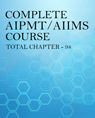Click to Chat

1800-1023-196

+91-120-4616500

CART 0

• 0

MY CART (5)

Use Coupon: CART20 and get 20% off on all online Study Material

ITEM
DETAILS
MRP
DISCOUNT
FINAL PRICE
Total Price: Rs.

There are no items in this cart.
Continue Shopping
`        Please help how to solve this problem...calorimetry problem....`
one year ago

```							Density of block = 6000 kg/m^3Mass = 1.2Kg => Volume = 0.0002 m^3Buoyant force on block(Fb) = (Volume of block)× (density of water)× g=> Fb = 0.0002×1000×10 = 2N (appx)Weight of block = mg = 12N (appx)=> Net force on spring = mg-(Fb) = 10N (appx)or kx = 10 => x = 10÷200 = 0.05 MTherefore, elastic potential energy of spring = 0.5kx^2 = 0.5 × 200 × 0.05^2 = 0.25JGravitational potential energy of block = mgh = 1.2 × 10 × 0.4 = 4.8J=> Total energy = 5.05JNow, Total energy = Heat of system = Internal energy of block + Internal energy of water => 1.2 × 250 × ∆T + 0.26 × 4200 × ∆T = 5.05JTherefore, ∆T = 5.05 ÷ (300+1092) = 0.003 ℃ or 0.003 K (appx)
```
one year ago
Think You Can Provide A Better Answer ?

## Other Related Questions on AIIMS MBBS

View all Questions »### Course Features

• 731 Video Lectures
• Revision Notes
• Previous Year Papers
• Mind Map
• Study Planner
• NCERT Solutions
• Discussion Forum
• Test paper with Video Solution### Course Features

• 728 Video Lectures
• Revision Notes
• Test paper with Video Solution
• Mind Map
• Study Planner
• NCERT Solutions
• Discussion Forum
• Previous Year Exam Questions